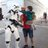# #379 – Using a Tooltip As a Magnifier

You can use a tooltip as a magnifier, so that when you hover over a control, it shows an enlarged view of the control.We define a tooltip to contain a Rectangle that is exactly twice the size of the associated control (using data binding and a value converter).  We then fill the Rectangle with a VisualBrush and bind the Visual property of the VisualBrush to the element that is hosting the tooltip.

```    <Window.Resources>
<loc:DoubleIntConverter x:Key="doubleIntConverter"/>

<ToolTip x:Key="reflectingTooltip" DataContext="{Binding Path=PlacementTarget, RelativeSource={x:Static RelativeSource.Self}}"
Placement="Center">
<Rectangle Width="{Binding ActualWidth, Converter={StaticResource doubleIntConverter}}"
Height="{Binding ActualHeight, Converter={StaticResource doubleIntConverter}}">
<Rectangle.Fill>
<VisualBrush Visual="{Binding}"/>
</Rectangle.Fill>
</Rectangle>
</ToolTip>
</Window.Resources>
```

Using the tooltip:

```        <Label Content="Can you read me now?" ToolTip="{StaticResource reflectingTooltip}"
HorizontalAlignment="Center"/>
```

The implementation of the value converter is left as an exercise.About Sean
1.Raul Bastian (@raulbastian) says:
•Sean says: